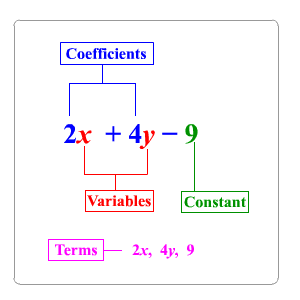Parts of an Expression

Algebraic expressions are combinations of variables , numbers, and at least one arithmetic operation.

For example, $2x+4y-9$ is an algebraic expression.Term: Each expression is made up of terms. A term can be a signed number, a variable, or a constant multiplied by a variable or variables.

Factor: Something which is multiplied by something else. A factor can be a number, variable, term, or a longer expression. For example, the expression $7x\left(y+3\right)$ has three factors: $7$ , $x$ , and $\left(y+3\right)$ .

Coefficient: The numerical factor of a multiplication expression that contains a variable. Consider the expression in the figure above, $2x+4y-9$ . In the first term, $2x$ , the coefficient is $2$ : in the second term, $4y$ , the coefficient is $4$ .

Constant: A number that cannot change its value. In the expression $2x+4y-9$ , the term $9$ is a constant.

Like Terms: Terms that contain the same variables such as $2m$ , $6m$ or $3xy$ and $7xy$ . If an expression has more than one constant terms, those are also like terms.

 Expression Word Phrases $n+5$ Sum of a number and $5$ $m-7$ Difference of a number and $7$ $6x$ Product of $6$ and a number $y÷9$ Quotient of a number and $9$

Example:

Identify the terms, like terms, coefficients, and constants in the expression.

$9m-5n+2+m-7$

First, we can rewrite the subtractions as additions.

$9m-5n+2+m-7=9m+\left(-5n\right)+2+m+\left(-7\right)$

So, the terms are $9m$ , $\left(-5n\right)$ , $m$ , $2$ , and $\left(-7\right)$ .

Like terms are terms that contain the same variables.

$9m$ and $9m$ are a pair of like terms . The constant terms $2$ and $-7$ are also like terms.

Coefficients are the numerical parts of a term that contains a variable.

So, here the coefficients are $9$ , $\left(-5\right)$ , and $1$ . ( $1$ is the coefficient of the term $m$ .)

The constant terms are the terms with no variables, in this case $2$ and $-7$ .

Algebraic expressions must be written and interpreted carefully. The algebraic expression $5\left(x+9\right)$ is not equivalent to the algebraic expression, $5x+9$ .

See the difference between the two expressions in the table below.

 Word Phrases Algebraic Expression Five times the sum of a number and nine $5\left(x+9\right)$ Nine more than five times a number $5x+9$

In writing expressions for unknown quantities, we often use standard formulas. For example, the algebraic expression for "the distance if the rate is $50$ miles per hour and the time is $T$ hours" is $D=50T$ (using the formula $D=RT$ ).

An expression like ${x}^{n}$ is called a power. Here $x$ is the base, and $n$ is the exponent. The exponent is the number of times the base is used as a factor. The word phrase for this expression is " $x$ to the ${n}^{\text{th}}$ power."

Here are some of the examples of using exponents.

 Word Phrases Algebraic Expression Seven times $m$ to the fourth power $7{m}^{4}$ The sum of $x$ squared and $12$ times of $y$ ${x}^{2}+12y$ $x$ cubed times $y$ to the sixth power ${x}^{3}\cdot {y}^{6}$